## NCERT Chapter Solutions and Other Numerical Problems

www.eduvictors.comJoseph Fourier - How is he related to Dimensions?
Q1: Define the term 'Dimension'

Answer: The term 'dimension' is used to refer to the physical nature of a quantity and the type of unit used to specify it. Mathematically dimensions of a physical quantity are the powers to which the fundamental quantities must be raised.

e.g. Dimension of velocity = Displacement / time = [L]/[T] = [M0][L1][T-1]

Q2: What are dimensional constants?

Answer:  Constants which possess dimensions are called dimensional constants. E.g. Planck' Constant.

Q3: What are dimensional variables?

Answer: Those physical quantities which possess dimensions but do not have a fixed value are called dimensional variables. E.g. Displacement, Force, velocity etc.

Q4: What are dimensionless quantities?

Answer: Physical quantities which do not possess dimensions are called dimensionless quantities. E.g. Angle, specific gravity, strain. In general, physical quantity which is a ratio of  two quantities of same dimension will be dimensionless.

Q5: Define the principle of homogeneity of dimensions. On What principle is it based?

Answer: The principle of homogeneity of dimensions states that an equation is dimensionally correct if the dimensions of the various terms on either side of the equation are the same.

This principle is based on the fact that two quantities of the same dimension only can be added up, and the resulting quantity also possess the the same dimension.

i.e. In equation X + Y = Z is valid if the dimensions of X, Y and Z are same.

Q6: Who introduced Dimension Analysis

Answer: Fourier (Joseph Fourier - French Mathematician)

Q7: List the basic dimensions.
• Length - L
• Time - T
• Mass - M
• Temperature - K or  θ
• Current - A
Q8:  What are the uses (applications) of dimensional analysis?

Answer: The applications of dimensional analysis are:
1. To convert a physical quantity from one system of units to another.
2. To check the dimensional correctness of a given equation.
3. establish a relationship between different physical quantities in an equation.
Q9 (NCERT): A book with many printing errors contains four different formulas for the displacement
y of a particle undergoing a certain periodic motion:

(a) y = a sin 2π t/T
(b) y = a sin vt
(c) y = (a/T) sin t/a
(d) y = (a 2) (sin 2πt / T + cos 2πt / T )

(a = maximum displacement of the particle, v = speed of the particle. T = time-period
of motion). Rule out the wrong formulas on dimensional grounds.

Given,
Dimension  of a = displacement = [M0L1T0]
Dimension of v (speed) =  distance/time = [M0L1T-1]
Dimension of  t or T (time period) = [M0L0T1]

Trigonometric function sine is a ratio, hence it must be dimensionless.

(a)  y = a sin 2π t/T (correct )
Dimensions of RHS =  [L1] sin([T].[T-1] ) =  [M0L1T0] = LHS  (eqation is correct).

(b) y = a sin vt  (wrong )
RHS =  [L1] sin([LT-1] [T1]) =  [L1] sin([L]) = wrong, since trigonometric function must be dimension less.

(c) y = (a/T) sin t/a (wrong )
RHS = [L1] sin([T].[L-1] ) = [L1] sin([TL-1] ) = wrong, sine function must be dimensionless.

(d)  y = (a 2) (sin 2πt / T + cos 2πt / T )  (correct )
RHS =  [L1] ( sin([T].[T-1] + cos([T].[T-1] ) = [L1] ( sin(M0L1T0) + cos(M0L1T0) )
= [L1]  = RHS  = equation is dimensionally correct.

Q10(NCERT): A famous relation in physics relates ‘moving mass’ m to the ‘rest mass’ mo of a particle in terms of its speed v and the speed of light, c. (This relation first arose as a consequence of special relativity due to Albert Einstein). A boy recalls the relation almost correctly but forgets where to put the constant c. He writes
Dimension of m (mass) = [M1L0T0]
Dimension of m0 (mass) = [M1L0T0]

Dimension of v (velocity) = [M0L1T-1]

∴ Dimension of v2 = [M0L2T-2]

Dimension of c (velocity) = [M0L1T-1]

Applying principle of homogeneity of dimensions,  [LHS] = [RHS] = [M1L0T0]
⇒ The equation (1- v2)½  must be dimension less, which is possible if we have the expressions as:
(1 -  v2/c2) The equation after placing 'c' will be:

Q11: Check the following equation for calculating displacement is dimensionally correct or not
(a) x = x0 + ut + (1/2) at2
where, x is displacement at given time t
xo is the displacement at t = 0
u is the velocity at t = 0
a represents the acceleration.

(b) P = (ρgh)½
where P is the pressure,
ρ is the density
g is gravitational acceleration
h is the height.

(a)  x = x0 + ut + (1/2) at2
Applying principle of homogeneity, all the sub-expressions  of the equation must have the same dimension and be equal to [LHS]
Dimension of  x = [M0L1T0]

Dimensions of  sub-expressions of [RHS] must be [M0L1T0]
⇒ Dimension of  x0 (displacement) = [M0L1T0] = [LHS]

Dimension of ut  = velocity x time = [M0L1T-1][M0L0T1] = [M0L1T0] = [LHS]

Dimension of  at2 = acceleration x (time)2  = [M0L1T-2][M0L0T-2] = [M0L1T0] = [LHS]

∴ The equation is dimensionally correct.

(b) P = (ρgh)½

Dimensions of LHS i.e. Pressure [P] = [M1L-1T-2]

Dimensions of ρ = mass/volume =  [M1L-3T0]
Dimensions of g (acceleration) = [M0L1T-2]
Dimensions of h (height) = [M0L1T0]

Dimensions of RHS = [(ρgh)½] = ([M1L-3T0]. [M0L1T-2].[M0L1T0])½ = ([M1L-1T-2])½
= [M½LT-1]  ≠ [LHS]
The given equation is not dimensionally correct.Q12: Prove that trigonometric function like sin θ are dimensionless.

Answer:  As shown in figure, sin θ = y/r. = [L]/[L] = 1
⇒ sin θ is dimensionless

Q13 (NCERT): A man walking briskly in rain with speed v must slant his umbrella forward making an angle θ with the vertical. A student derives the following relation between θ and v : tan θ = v and checks that the relation has a correct limit: as v → 0, θ →0, as expected. (We are assuming there is no strong wind and that the rain falls vertically for a stationary man). Do you think this relation can be correct ? If not, guess the correct relation.

Answer: Given, v = tanθ
Dimensions of  LHS = [v] = [M0L1T-1]
Dimension of  RHS = [tanθ] = [M0L0T0]      (trigonometric ratios are dimensionless)

Since [LHS] ≠ [RHS]. Equation is dimensionally incorrect.

To make the equation dimensionally correct, LHS should also be dimension less. It may be possible if consider speed of rainfall (Vr) and the equation will become:
tan θ = v/Vr

Q14: Hooke’s law states that the force, F, in a spring extended by a length x is given by F = −kx.
According to Newton’s second law F = ma, where m is the mass and a is the acceleration.
Calculate the dimension of the spring constant k.

Answer: Given, F = -kx
⇒ k = - F/x

F = ma, the dimensions of force is:
[F] = ma = [M1L0T0].[M0L1T-2] = [M1L1T-2]
Therefore, dimension of spring constant (k) is:
[k] = [F]/[x] = [M1L1T-2].[M0L-1T0] = [M1L0T-2] or [MT-2] .....     (answer)

Q15: Compute the dimensional formula of electrical resistance (R).

Answer: According to Ohm's law
V = IR or R = V/I
Since Work done (W) = QV  where Q is the charge
⇒ R = W/QI = W/I2t                           (I = Q/t)

Dimensions of Work [W] = [M1L2T-2]
∴Dimension of R =  [R] = [M1L2T-2][A-2T-1] = [M1L2T-3A-2]   ... (answer)

Note: Formula used to convert physical quantity from one system of units to another.
If n1 and n2 are numerical units of a physical quantities and U1 and U2 represent different system units, thenQ16: Convert 76 cm of mercury pressure into N m-2 using the dimensional formula. (Density of Hg = 13.6 gm/mL)

Pressure P = hdg            (h = height, d = density, g = acceleration due to gravity).
In cgs system, 76 cm of Hg will exert pressure (P1), i.e.
P1 = 76 x 13.6 x 980 = 1012928 dynes cm-2.

Dimension of Pressure in [M1L-1T-2]
Applying to the formula (shown above in note), a = 1, b = -1 and c = -2

Q17: A calorie is a unit of heat or energy and it equals about 4.2 J where 1J = 1 kg m2 s–2. Suppose we employ a system of units in which the unit of mass equals α kg, the unit of length equals β m, the unit of time is γ s. Show that a calorie has a magnitude 4.2 α–1 β–2 γ2 in terms of the new units.

Answer:  Considering the unit conversion formula,
n1U1 = n1U2
n1[M1aL1bT1c] = n2[M2aL2bT2c]
Given here,  1 Cal = 4.2 J = 4.2  kg m2 s–2.

n1 = 4.2,  M1 = 1kg,  L1 = 1m,  T1 = 1 sec
and
n2 = ?,  M2 = α kg,  L2 = βm,  T2 = γ sec

The dimensional formula of energy is =  [M1L2T-2]
⇒ a = 1, b =1 and c = -2  Putting these values in above equation,

n2= n1[M1/M2]a[L1/L2]b[T1/T2]c

= n1[M1/M2]1[L1/L2]2[T1/T2]-2

= 4.2[1Kg/α kg]1[1m/βm]2[1sec/γ sec]-2 = 4.2 α–1 β–2 γ2              ... (answer)

Q18: The kinetic energy K of a rotating body depends on its moment of inertia I and its angular speed ω. Considering the relation to be K = kIaωb where k is dimensionless constant.
Find a and b. Moment of Inertia of a spehere about its diameter is (2/5)Mr2

Dimensions of Kinetic energy K = [M1L2T-2]
Dimensions of Moment of Inertia (I) = [ (2/5)Mr2] = [ML2T0]
Dimensions of angular speed ω = [θ/t] = [M0L0T-1]
Applying principle of homogeneity in dimensions in the equation K = kIaωb

[M1L2T-2] = k ( [ML2T0])a([M0L0T-1])b
[M1L2T-2] = k [MaL2aT-b]
⇒ a = 1 and b = 2
⇒ K = kIω2                                                          ... (answer)

Q19: What are the limitations of Dimensional Analysis?

Answer: Limitations of Dimensional Analysis are:
1. It cannot determine value of dimensionless constants.
2. We cannot use this method to equations involving exponential and trigonometric functions.
3. It cannot be applied to an equation involving more than three physical quantities.
4. It is a too not a solution i.e.  It can check only if the equation is dimensionally correct or not. But cannot say the equation is absolutely correct.

Q20: Convert 1 Newton into dyne using method of dimensions.

Answer: Dimensions of Force = [M1L1T-2]
Considering dimensional unit conversion formula i.e.  n1[M1aL1bT1c] = n2[M2aL2bT2c]
⇒ a = 1, b = 1 and c = -2
In SI system,  M1 = 1kg,        L1 = 1m   and T1 = 1s
In cgs system, M2 = 1g,    L2 = 1cm  and T2 = 1s

Putting the values in the conversion formula,
n2 = n1(1Kg/1g)1.(1m/1cm)1(1s/1s)-2 = 1.(103/1g)(102cm) = 105dyne   ...(answer)

Q21: The centripetal force  (F) acting on a particle (moving uniformly in a circle) depends on the mass (m) of the particle, its velocity (v) and radius (r) of the circle. Derive dimensionally formula for force (F).

Answer:  Given,   F ∝ ma.vb.rc
∴ F = kma.vb.rc      (where k is constant)

Putting dimensions of each quantity in the equation,

[M1L1T-2] = [M1L0T0]a. [M0L1T-1]b. [M0L1T0]= [MaLb+cT-b]

⇒  a =1, b +c = 1, -b = -2
⇒  a= 1, b = 2, c = -1
∴ F = km1.v2.r-1     =  kmv2/r

Q22: If the velocity of light c, gravitational constant G and planks constant h be chosen as fundamental units, find the value of a gram, a cm and a sec in term of new unit of mass, length and time respectively.
(Take c = 3 x 1010 cm/sec, G = 6.67 x 108 dyn cm2/gram2 and  h = 6.6 x 10-27 erg sec)

c = 3 x 1010 cm/sec
G = 6.67 x 108 dyn cm2/gm2
h = 6.6 x 10-27 erg sec
Putting respective dimensions,
Dimension formula for c = [M0L1T-1] = 3 x 1010 cm/sec                             .... (I)
Dimensions of G             =  [M-1L3T-2] = 6.67 x 108 dyn cm2/gm2                 ...(II)
Dimensions of h              = [M1L2T-1] =  6.6 x 10-27 erg sec                       ...(III)

(Note: Applying newton's law of gravitation, you can find dimensions of G i.e. G = Fr2/(mM)
Similarly, Planck's Constant (h) = Energy / frequency)
To get M, multiply eqn-I and III and divide by eqn.-II,
⇒ [M0L1T-1].[M1L2T-1].[M1L-3T2]
= ( 3 x 1010 cm/sec).( 6.6 x 10-27 erg sec)/ 6.67 x 108 dyn cm2/gm2
⇒[M2] = 2.968 x 10-9
⇒[M] =  0.5448 x 10-4 gm
or 1gm = [M]/0.5448 x 10-4 = 1.835 x 10-4 unit of mass

To obtain length [L],  eqn.-II x eqn-III / cube of eqn.-I i.e.
[M-1L3T-2].[M1L2T-1].[M0L-3T3]
= (6.67 x 108 dyn cm2/gm2 ).( 6.6 x 10-27 erg sec)/(3 x 1010 cm/sec)3

⇒ [L2] =  1.6304 x 10-65 cm2
[L]  =  0.4038 x 10-32 cm
or  1cm = [L]/ 0.4038 x 10-32 = 2.47 x 10-32unit of length

In eqn-I,  [M0L1T-1] = 3 x 1010 cm/sec
⇒  [T] = [L] ÷ 3 x 1010cm/s
⇒  [T] =  0.4038 x 10-32 cm  ÷ 3 x 1010cm/s = 0.1345 x 10-42 s
or 1s = [T]/0.1345 x 10-42 s = 7.42 x 1042 unit of time

Q 23: A student while doing an experiment finds that the velocity of an object varies with time and it can be expressed as equation:
v = Xt2 + Yt +Z .
If units of v and t are expressed in terms of SI units, determine the units of constants X, Y and Z in the given equation.

Answer: Given,  v = Xt2 + Yt +Z
Dimensions of velocity v =  [M0L1T-1]
Applying applying principle of homogeneity in dimensions, terms must have same dimension.
[v] = [Xt2] + [Yt] + [Z]
∴ [v] = [Xt2]
⇒ [X] = [v] /[t2]  =  [M0L1T-1] / [M0L0T2] = [M0L1T-3]              ....(i)

Similarly, [v] = [Yt]
⇒  [Y] = [v] / [t] = [M0L1T-1]/ [M0L0T-1] = [M0L1T-2]             ...(ii)

Similarly, [v]= [Z]
[Z] =  [M0L1T-1]      ...(iii)

Unit of X = m-s-3
Unit of Y = m-s-2
Unit of Z = m-s-1

Q24: Express Capacitance in terms of dimensions of fundamental quantities i.e. Mass (M), Length(L), Time(T) and Ampere(A)

Answer: Capacitance(C) is defined as the ability of a electric body to store electric charge.
∴ Capacitance (C) = Total Charge(q) / potential difference between two plates (V)
= Coulomb/ Volt
∵  Volt = Work done (W)/ Charge(q) = Joule/Coulomb
⇒  Capacitance (C) = Charge(q)2/ Work(W)
∵ Charge (q) = Current (I) × Time(t)
Dimension of [q] = [AT]                                                 ----------- (I)
Dimension of Work = Force × distance = [MLT-2][L] = [ML2T-2]            --------- (II)

Putting values of I and II,
[C] = ([AT])2/ [ML2T-2] = [M-1L-2T2+2A2] = [M-1L-2T4A2]

Physical Quantities having the same dimensional formula:
a. impulse and momentum.
b. force, thrust.
c. work, energy, torque, moment of force, energy
d. angular momentum, Planck’s constant, rotational impulse
e. force constant, surface tension, surface energy.
f. stress, pressure, modulus of elasticity.
g. angular velocity, frequency, velocity gradient
h. latent heat, gravitational potential.
i. thermal capacity, entropy, universal gas constant and Boltzmann’s constant.
j. power, luminous flux.

Q25: If Force (F), velocity (V) and acceleration (A) are taken as the fundamental units instead of mass, length and time, express pressure and impulse in terms of F, V and A.

Answer: We know that Force = mass ✕ acceleration
⇒ mass = FA-1
and  length = velocity ✕ time = velocity ✕  velocity ÷  acceleration = V2A-1
and time = VA-1

∵ Pressure = Force  ÷ Area = F ÷ (V2A-1)2 = FV-4A2
Impulse = Force ✕ time = FVA-11.very nyc....

2.Amazing and very useful questions...

3.INTERSTING QUESTION

4.useful questions

5.6.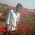Its so helping

7.your explanations helps a lot in understanding the ideology and method of solution...

8.thanx for such a great information .this is really going to help in my upcomming final exams.thanks a lot again.

9.amazing'''',,,, my doubts are cleared now
thank you very much

10.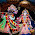thnks very much to cbse .its vrry nice

11.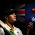tqs once again

12.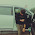pls sugest method.

13.if force depends on masses m1, m2 and distance between masses i.e. r than how do we find dimension formulae of force?

14.why we not use degree symbol in kelvin temparature representation?

15.will u plzz solve ... y=asin(wt-kx)
where,,
y=displacement
a=amplitude
t=time &
x=distance

1.Since trigonometric functions does not have dimensions,so sin(wt-kx) would be cancel out.therefore,y=L and a=L,so it is dimensionally correct

2.please solve my problem:-
1.The position x of a particle at time t is given by
x=b/a(1-e^at) where a and b are non-zero constants.Find the dimension of b.

3.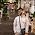16.To keep an object in a circle at constant speed requires a force called the centripetal force. Do a dimensional analysis of the centripetal force..

17.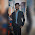Vry usful..question

18.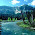19.but put different types of numericals modals

20.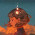21.Thank you but didn't understand 22^nd question😅

1.2.The 22nd questions can be solved in an easier way also. You can express the dimensions in terms of the given one by the 3rd law and take it as a,and c and then just multiply by the value . you will get the answer

22.Awesome n useful , but can improve by adding more infos

23.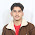A sphere of radius 50mm and mass 1kg falling vertically through air of density 1.2kg / m^3 attains a steady velocity of 11m / s .
If the above equation is applied to its fall what is the value of k in this case? Ans :2 21

24.A sphere of radius 50mm and mass 1kg falling vertically through air of density 1.2kg / m^3 attains a steady velocity of 11m / s .
If the above equation is applied to its fall what is the value of k in this case? Ans :2 21

25.26.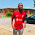27.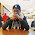God bless you. Thanks for these useful data :)

28.plz solve this problem the force acting on the body depends upon the mass m of the body acceleration find the expression of the body for the forc using the dimensional formula

1.According to question For(force) depends on mass and acceleration.F=kma where k is constant. Now solving RHS

m= [M^1] and acceleration is distance/time^2. Therefore F = [M][L/T^2] therefore dimensional formula is [MLT^-2]. CHEERS ITS SOLVED.

29.